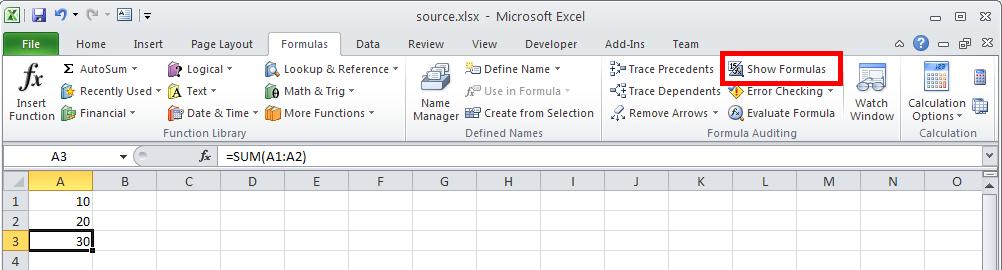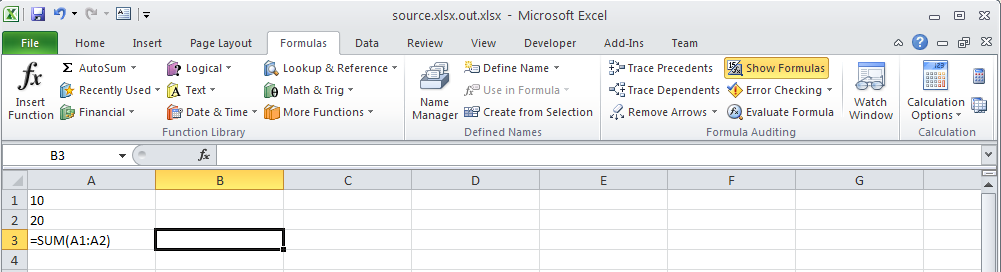# Show Formulas instead of Values in a Worksheet

Contents
[ ]

Aspose.Cells provides a Worksheet.setShowFormulas() property. Set this to true to set Microsoft Excel to display formulas.

The following image shows the worksheet which has a formula in cell A3: =Sum(A1:A2).

Worksheet with formula in cell A3The following image shows the formula instead of the calculated value, enabled by setting the Worksheet.setShowFormulas() property to true with Aspose.Cells.

Worksheet now shows formula instead of the calculated value